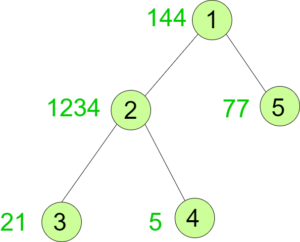# Count of Nodes which has Prime Digit sum weight in a Tree

Given a weighted tree, the task is to count the number of nodes whose sum of digits of weights is a Prime Number.

Examples:

Input:Output: 2
Explanation:
Node 1: digitSum(144) = 1 + 4 + 4 = 9
Node 2: digitSum(1234) = 1 + 2 + 3 + 4 = 10
Node 3: digitSum(21) = 2 + 1 = 3
Node 4: digitSum(5) = 5
Node 5: digitSum(77) = 7 + 7 = 14
Only the sum of digits of the weights of nodes 3 and 4 are prime.

Approach: To solve the problem mentioned above we have to perform DFS on the tree and for every node and check if the sum of the digits of its weight is prime or not. If yes then increment the count. To check if the digit sum is prime or not, we will use Sieve Of Eratosthenes. Create a sieve which will help us to identify if the degree is prime or not in O(1) time.

Below is the implementation of the above approach:

 `// C++ program to Count Nodes ` `// which has Prime Digit ` `// Sum Weight in a Tree ` ` `  `#include ` `using` `namespace` `std; ` ` `  `int` `MAX = 1000000; ` `int` `ans = 0; ` ` `  `vector<``int``> graph; ` `vector<``int``> weight(100); ` ` `  `// Function to create Sieve ` `// to check primes ` `void` `SieveOfEratosthenes( ` `    ``bool` `prime[], ``int` `p_size) ` `{ ` `    ``// false here indicates ` `    ``// that it is not prime ` `    ``prime = ``false``; ` `    ``prime = ``false``; ` ` `  `    ``for` `(``int` `p = 2; p * p <= p_size; p++) { ` ` `  `        ``// If prime[p] is not changed, ` `        ``// then it is a prime ` `        ``if` `(prime[p]) { ` ` `  `            ``// Update all multiples of p, ` `            ``// set them to non-prime ` `            ``for` `(``int` `i = p * 2; ` `                 ``i <= p_size; ` `                 ``i += p) ` `                ``prime[i] = ``false``; ` `        ``} ` `    ``} ` `} ` ` `  `// Function to return the ` `// sum of the digits of n ` `int` `digitSum(``int` `n) ` `{ ` `    ``int` `sum = 0; ` `    ``while` `(n) { ` `        ``sum += n % 10; ` `        ``n = n / 10; ` `    ``} ` `    ``return` `sum; ` `} ` ` `  `// Function to perform dfs ` `void` `dfs(``int` `node, ` `         ``int` `parent, ` `         ``bool` `prime[]) ` `{ ` `    ``// If sum of the digits ` `    ``// of current node's weight ` `    ``// is prime then increment ans ` `    ``int` `sum = digitSum(weight[node]); ` `    ``if` `(prime[sum]) ` `        ``ans += 1; ` ` `  `    ``for` `(``int` `to : graph[node]) { ` `        ``if` `(to == parent) ` `            ``continue``; ` `        ``dfs(to, node, prime); ` `    ``} ` `} ` ` `  `// Driver code ` `int` `main() ` `{ ` ` `  `    ``// Weights of the node ` `    ``weight = 144; ` `    ``weight = 1234; ` `    ``weight = 21; ` `    ``weight = 5; ` `    ``weight = 77; ` ` `  `    ``// Edges of the tree ` `    ``graph.push_back(2); ` `    ``graph.push_back(3); ` `    ``graph.push_back(4); ` `    ``graph.push_back(5); ` ` `  `    ``bool` `prime[MAX]; ` `    ``memset``(prime, ``true``, ``sizeof``(prime)); ` ` `  `    ``SieveOfEratosthenes(prime, MAX); ` ` `  `    ``dfs(1, 1, prime); ` ` `  `    ``cout << ans; ` ` `  `    ``return` `0; ` `} `

Output:

```2
```My Personal Notes arrow_drop_upCheck out this Author's contributed articles.

If you like GeeksforGeeks and would like to contribute, you can also write an article using contribute.geeksforgeeks.org or mail your article to contribute@geeksforgeeks.org. See your article appearing on the GeeksforGeeks main page and help other Geeks.

Please Improve this article if you find anything incorrect by clicking on the "Improve Article" button below.